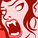Announcements
Applications are open for the 2024 Qlik Luminary Program. Apply by December 15 here.
cancel
Showing results for
Did you mean:Contributor III

## calculation with tables

Hey there,

I'm more or less new and have a hopefully pretty simple quetsion.

I have a basic table (step one) which contains data of earnings and costs. The values in this table are all positve.

These 4 accounts can also be grouped as "profit contribution". Of course, this is earnings-costs. So i wrote a statement in step two in the set-analysis ( If([Account type] ='costs', Sum(Value)*-1,sum(Value)) )....at first, it seems to work just fine, because the value 25 is now -25.

however, when trying to aggregate it by only showing "profit contribution" it still gives me the wrong value.

Can I solve this problem by any formula in the set analysis or do I have to do the value*-1-calculation in the scripting.Labels (1)
• ### profit and loss calculation in simple tables

1 Solution

Accepted SolutionsMVP

Try this

``````RangeSum(
Sum({<[Account type] = {'costs'}>} Value)*-1,
Sum({<[Account type] = {'earnings'}>} Value)
)``````
3 RepliesMVP

Try this

``````RangeSum(
Sum({<[Account type] = {'costs'}>} Value)*-1,
Sum({<[Account type] = {'earnings'}>} Value)
)``````Contributor III
Author

Many thanks, works perfectly fine!!

I just tried

Sum(If([Account type]='costs',Value*-1,Value))

which also kind of works 🙂

THanks Sunny_talwar :)!Partner - Specialist

It looks like you are doing a financial report and you will likely be adding in hundreds of accounts. I would suggest creating a table in the load script that would allow you to set the sign for each account. You might need to create different columns for other measures depending on how they are handled. then your expressions in your chart can be something like

``````//Put this in your load script
AccountMultipliers: